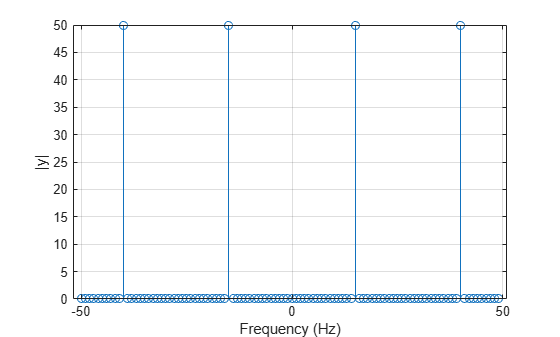# angle

## Syntax

theta = angle(z)

## Description

example

theta = angle(z) returns the phase angle in the interval [-π,π] for each element of a complex array z. The angles in theta are such that z = abs(z).*exp(i*theta).

## Examples

collapse all

Create a complex number, and compute its magnitude and phase.

z = 2*exp(i*0.5)
z = 1.7552 + 0.9589i 
r = abs(z)
r = 2 
theta = angle(z)
theta = 0.5000 

Create a signal that consists of two sinusoids of frequencies 15 Hz and 40 Hz. The first sinusoid has a phase of $-\pi /4$, and the second has a phase of $\pi /2$. Sample the signal at 100 Hz for one second.

fs = 100; t = 0:1/fs:1-1/fs; x = cos(2*pi*15*t - pi/4) - sin(2*pi*40*t);

Compute the Fourier transform of the signal. Plot the magnitude of the transform as a function of frequency.

y = fft(x); z = fftshift(y); ly = length(y); f = (-ly/2:ly/2-1)/ly*fs; stem(f,abs(z)) xlabel 'Frequency (Hz)' ylabel '|y|' gridCompute the phase of the transform, removing small-magnitude transform values. Plot the phase as a function of frequency.

tol = 1e-6; z(abs(z) < tol) = 0; theta = angle(z); stem(f,theta/pi) xlabel 'Frequency (Hz)' ylabel 'Phase / \pi' grid## Input Arguments

collapse all

Input array, specified as a scalar, vector, matrix, or multidimensional array. When the elements of z are non-negative real numbers, angle returns 0. When the elements of z are negative real numbers, angle returns π.

Data Types: double | single
Complex Number Support: Yes

## Algorithms

angle takes a complex number z = x + iy and uses the atan2 function to compute the angle between the positive x-axis and a ray from the origin to the point (x,y) in the xy-plane.

## Version History

Introduced before R2006a